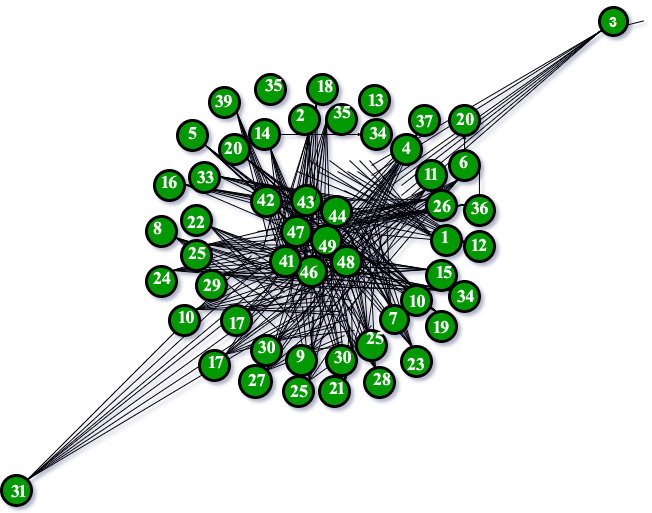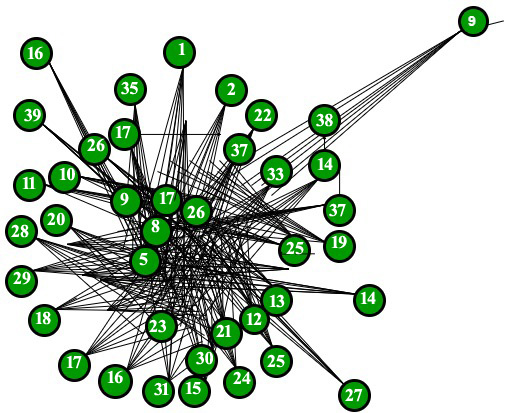# Barabasi Albert Graph (for Scale Free Models)

• Last Updated : 15 Nov, 2021

The current article would deal with the concepts surrounding the complex networks using the python library Networkx. It is a Python language software package for the creation, manipulation, and study of the structure, dynamics, and function of complex networks. With NetworkX you can load and store networks in standard and nonstandard data formats, generate many types of random and classic networks, analyze network structure, build network models, design new network algorithms, draw networks, and much more.

The current article would deal with the algorithm for generating random scale free networks for using preferential attachment model. The reason of interest behind this model dates back to the 1990s when Albert Lazlo Barabasi and Reka Albert came out with the path breaking research describing the model followed by the scale free networks around the world. They suggested that several natural and human-made systems, including the Internet, the World Wide Web, citation networks, and some social networks are thought to be approximately scale-free networks.
A scale-free network is a network whose degree distribution follows a power law, at least asymptotically. That is, the fraction P (k) of nodes in the network having k connections to other nodes goes for large values of k as

Attention reader! Don’t stop learning now. Get hold of all the important DSA concepts with the DSA Self Paced Course at a student-friendly price and become industry ready.  To complete your preparation from learning a language to DS Algo and many more,  please refer Complete Interview Preparation Course.

In case you wish to attend live classes with experts, please refer DSA Live Classes for Working Professionals and Competitive Programming Live for Students.Whereis a parameter whose value is typically in the range 2 << 3, although occasionally it may lie outside these bounds and c is a proportionality constant.

The Barabási–Albert model is one of several proposed models that generate scale-free networks. It incorporates two important general concepts: growth and preferential attachment. Both growth and preferential attachment exist widely in real networks. Growth means that the number of nodes in the network increases over time.
Preferential attachment means that the more connected a node is, the more likely it is to receive new links. Nodes with higher degree have stronger ability to grab links added to the network. Intuitively, the preferential attachment can be understood if we think in terms of social networks connecting people. Here a link from A to B means that person A "knows" or "is acquainted with" person B. Heavily linked nodes represent well-known people with lots of relations. When a newcomer enters the community, s/he is more likely to become acquainted with one of those more visible people rather than with a relative unknown. The BA model was proposed by assuming that in the World Wide Web, new pages link preferentially to hubs, i.e. very well-known sites such as Google, rather than to pages that hardly anyone knows. If someone selects a new page to link to by randomly choosing an existing link, the probability of selecting a particular page would be proportional to its degree.

Following image will describe the BA Model graph with 50 nodes following the preferential attachment model.The above graph completely satisfies the logic of the rich getting richer and the poor getting poorer.

Code:
The following code is a part of the function which we will eventually implement using the networkx library.

 def barabasi_albert_graph(n, m, seed=None):    """Returns a random graph according to the Barabási–Albert preferential    Attachment model.      A graph of n nodes is grown by attaching new nodes each with m    Edges that are preferentially attached to existing nodes with high degree.      Parameters    ----------    n : int        Number of nodes    m : int        Number of edges to attach from a new node to existing nodes    seed : int, optional        Seed for random number generator (default=None).      Returns    -------    G : Graph      Raises    ------    NetworkXError        If m does not satisfy 1 <= m < n.            if m < 1 or  m >=n:        raise nx.NetworkXError("Barabási–Albert network must have m >= 1"                               " and m < n, m = %d, n = %d" % (m, n))    if seed is not None:        random.seed(seed)      # Add m initial nodes (m0 in barabasi-speak)    G=empty_graph(m)    G.name="barabasi_albert_graph(%s,%s)"%(n,m)    # Target nodes for new edges    targets=list(range(m))    # List of existing nodes, with nodes repeated once for each adjacent edge    repeated_nodes=[]    # Start adding the other n-m nodes. The first node is m.    source=m    while source

The above code is a part of the networkx library which is used to handle the random graphs efficiently in python. One will have to install it before running the following code.

 >>> import networkx as nx>>> G= nx.barabasi_albert_graph(50,40)>>> nx.draw(G, with_labels=True)

To display the above graph, I used the matplotlib library. We need to install it before the execution of the below codes.

 >>> import matplotlib.pyplot as plt>>> plt.show()

So the final code seemed like:

 >>> import networkx as nx>>> import matplotlib.pyplot as plt  >>> G= nx.barabasi_albert_graph(40,15)>>> nx.draw(G, with_labels=True)>>> plt.show()`

Output:BA model for 40 nodes

Thus I would further like to describe more about the networkx library and it modules basically focusing on the centrality measure of a network (especially the scale free models).

References